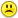+0

# In poker, a 5-card hand is called two pair if there are two cards of one rank, two cards of another rank, and a fifth card of a third rank.

0
3318
14

In poker, a 5-card hand is called two pair if there are two cards of one rank, two cards of another rank, and a fifth card of a third rank. (For example, QQ668 is two pair.) The order of the cards doesn't matter (so, for example, QQ668 and 68QQ6 are the same). How many 5-card hands are two pair? (Assume a standard 52-card deck with 13 ranks in each of 4 suits.)

Mar 14, 2015

#11
+5

This refers to distinct hands....."Distinct Hands" is the number of different ways to draw the hand, not counting different suits

For instance.....there is 1 "distinct" royal flush......but there are  4 suits of them......

Clearly....there are WAY more than 858 two-pair hands......Mar 15, 2015

#1
+5

There are 13*3 possibilities for the first pair, leaving 12*3 possibilities for the 2nd pair, leaving 11*4 possibilities for the singleton.

So:  13*3*12*3*11*4 in total:

$${\mathtt{13}}{\mathtt{\,\times\,}}{\mathtt{3}}{\mathtt{\,\times\,}}{\mathtt{12}}{\mathtt{\,\times\,}}{\mathtt{3}}{\mathtt{\,\times\,}}{\mathtt{11}}{\mathtt{\,\times\,}}{\mathtt{4}} = {\mathtt{61\,776}}$$

.
Mar 14, 2015
#2
+5

This is easiest calculated by first choosing the ranks, and then choosing the cards within those ranks...

So.....

From 13 ranks, we want to choose any 2  =  C(13,2)

And from the two ranks, we want to choose any 2 cards from each = [C(4,2)]^2

And we want to choose one card from any of the remaining 11 ranks.....

So we have

C(13,2)[C(4,2)}^2 * C(11,1)C(4,1)  = 123,552Mar 15, 2015
#3
+5

Ok I'll give you another possibility,

there are 52 ways of choosing the first card

there are 3 ways of chooing its pair

there are 48 ways of choosing the next number

and 3 ways of choosing its pair

there are 44 ways of choosing the single card

so that is    52*3*48*3*44

$${\mathtt{52}}{\mathtt{\,\times\,}}{\mathtt{3}}{\mathtt{\,\times\,}}{\mathtt{48}}{\mathtt{\,\times\,}}{\mathtt{3}}{\mathtt{\,\times\,}}{\mathtt{44}} = {\mathtt{988\,416}}$$

this is the third in your multiple choice answers   LOL

Mar 15, 2015
#4
+5

Poker probabilities.....

http://en.wikipedia.org/wiki/Poker_probability

This is actually very interesting.....!!!Mar 15, 2015
#5
0

Thanks Chris - that would be a good site for us to study from I expect.So there are 858 distinct possibilities.

Maybe this is interpreted differently from how I interpreted it.

I interpreted there being 4*3=12 ways of choosing 2 of any individual rank.

I wonder if I was suppose to think of all those as the same?

Mmm this definitely requires more study and consideration.        ヽ(•́o•̀)ノ

Mar 15, 2015
#6
+5

Sorry, Melody...I believe this question  asks how many two-pair hands are possible in a draw of 5 cards....if I'm interepreting this correctly.....I think my answer is the only correct one....

Look at the number of possible royal flushes in the table......4....!!!Mar 15, 2015
#7
0

doesn't that snip say that there are 858 possibilities?

Mar 15, 2015
#8
+5

Check the probability.....  123552/ 2598560   = about 4.75%

This says out of 2,598,560 possible hands, there are 123,552 hands consisting of two pairs....Mar 15, 2015
#9
0

so what is the 858 for?

Mar 15, 2015
#10
0

One thing about probabiliity is that it can be really difficult to interprete what you are being asked for.

The other thing about prob is that although you may be able to understand the correct answer when it is presented to you, you often still cannot understand why your own original answer is incorrect!

It is an interesting and a frustrating feild of methematics.------------------------------

I'm listening to this

Something different for you ChrisMar 15, 2015
#11
+5

This refers to distinct hands....."Distinct Hands" is the number of different ways to draw the hand, not counting different suits

For instance.....there is 1 "distinct" royal flush......but there are  4 suits of them......

Clearly....there are WAY more than 858 two-pair hands......CPhill Mar 15, 2015
#12
0

I don't understand.Maybe it is just too late for me.

When this music finishes I am off to bed.  :)

Mar 15, 2015
#13
0

$$\text{First we decide what ranks we will use. Then we will pick suits for all of the cards. We choose the two paired ranks in {13\choose 2}=78 ways and the remaining rank in {11\choose 1}=11 ways. Then we choose the suits for these cards in {4\choose 2}{4\choose 2}{4\choose 1}=144 ways. This gives a total of 78\cdot 11\cdot 144 = \boxed{123552} two pair hands.}$$

.
Mar 4, 2017
#14
0

$$\text{First we decide what ranks we will use. Then we will pick suits for all of the cards.} \text{We choose the two paired ranks in {13\choose 2}=78 ways and the remaining rank in {11\choose 1}=11 ways.} \text{Then we choose the suits for these cards in {4\choose 2}{4\choose 2}{4\choose 1}=144 ways.} \text{This gives a total of 78\cdot 11\cdot 144 = \boxed{123552} two pair hands.}$$

.
Mar 4, 2017